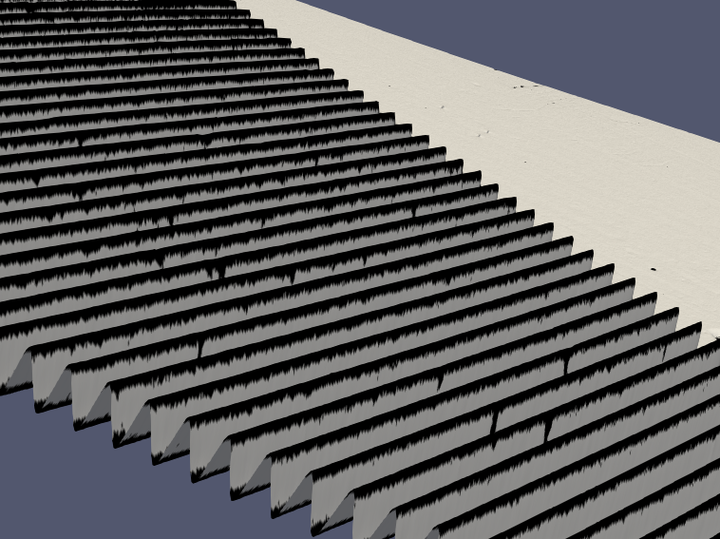# Low Contrast

## 1. Motivation

Since the intensity depends of the phase except when the reflection of the screen goes outside of the camera, points on the surface that have an phase-independent intensity (meaning a low contrast) are corrupted and not reliable.

Moreover, a rough surface will make the camera pixel to see multiple screen pixels by reflexion, making once again the intensity phase-independent and the contrast low.

## 2. Threshold computation

A threshold allows us to tell whether a point has a low contrast or not.

Soit $C = \frac{C_x + C_y}{2}$ the mean contrast map along x and y. We consider the simplified model :

$I_1 = I_0 \times R$
$I_n = I_{n-1} \times R = ... = I_0 R^n$

Where $I_0$ is the incident ray, $I_i$ is the intensity of the ray reflected $i$ times and $R\in (0,1)$ is the reflectivity of the surface. We consider R as a constant along x and along y depending only of the material in which the surface is made.

We can estimate this reflectivity by computing the mean value of contrast map.

$\overline{R_0} = mean(C)$

Since $0 < R < 1$, we have that $I_{n>2} < I_2$, so we can create a first threshold $R'$ that will detect even higher number of reflections :

$R' = \frac{\overline{R_0}^2 + \overline{R_0}}{2}$

Noting that the estimation of the reflectivity of the surface was made with the corrupted low contrast data we are trying to exclude, the threshold is corrupted too. We can improve the precision of that threshold by recomputing it without these rought selecting of low contrast area.

$\overline{R_1} = mean( C \setminus \{c_{ij} | c_{ij} < R' \})$

We get the improved threshold

$R'' = \frac{\overline{R_1}^2 + \overline{R_1}}{2}$

## 3. Implementation

``````image_t Dcontrast_ = image_t::Zero( nrows, ncols ); (1)

image_t cxy = (cx.array()+cy.array())/2; (2)

auto r = cxy.mean();
auto R = ( r*r + r )/2.; (3)

auto cxy_row { cxy.reshaped() };
std::sort( cxy_row.begin(), cxy_row.end() );
auto med = cxy_row.size() % 2 == 0 ?
cxy_row.segment( (cxy_row.size()-2)/2, 2 ).mean() :
cxy_row( cxy_row.size()/2 ); (4)

cxy = (cx.array()+cy.array())/2; (5)

image_t greaterThanR = (cxy.array() > R).select(cxy, matrix_1.array()*med); (6)

r = greaterThanR.mean();
R = ( r*r + r )/2.; (7)

Dcontrast_ = (cxy.array() > R).select(matrix_1, matrix_0); (8)``````
 1 Construction of a map with 0 everywhere 2 Mean of horizontal and vertical contrast for each point 3 First threshold computed with every data even corrupted one 4 Compute the median of every coefficient of the matrix 5 Un-sorting the contrast map wich was being sorted by the computation of the contrast mean value. 6 Rough selection to make a second threshold without the corrupted data (replacing potentially corrupted data by the median value) 7 Second threshold not corrupted by corrupted data 8 Final selection

## 4. Results

Low Contrast resultsThe Etalon is known to have a low contrast on the top and bottom of its waves.
• Histogram